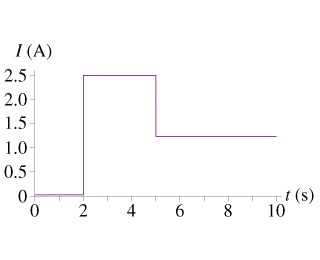# Problem: The graph in the figure shows the current through a 2.0 ohm resistor as a function of time.What was the total energy dissipated by the resistor during this time?

###### FREE Expert Solution

Power in circuits:

$\overline{){\mathbf{P}}{\mathbf{=}}{\mathbf{V}}{\mathbf{i}}{\mathbf{=}}{{\mathbf{i}}}^{{\mathbf{2}}}{\mathbf{R}}{\mathbf{=}}\frac{{\mathbf{V}}^{\mathbf{2}}}{\mathbf{R}}}$

Energy:

$\overline{){\mathbf{E}}{\mathbf{=}}{\mathbf{P}}{\mathbf{t}}}$

E1 ( 0s - 2s)

80% (323 ratings)###### Problem Details

The graph in the figure shows the current through a 2.0 ohm resistor as a function of time.What was the total energy dissipated by the resistor during this time?